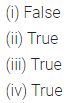# Selina Concise Mathematics Class 6 ICSE Solutions Chapter 9 Playing with Numbers

## Selina Concise Mathematics Class 6 ICSE Solutions Chapter 9 Playing with Numbers

Selina Publishers Concise Mathematics Class 6 ICSE Solutions Chapter 9 Playing with Numbers

### Playing with Numbers Exercise 9A – Selina Concise Mathematics Class 6 ICSE Solutions

(Using BODMAS)
Question 1.
19 – (1 + 5) – 3
Solution:Question 2.
30 x 6 + (5 – 2)
Solution: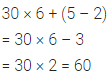Question 3.
28 – (3 x 8) + 6
Solution: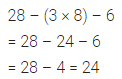Question 4.
9 – [(4 – 3) + 2 x 5]
Solution:Question 5.
[18 – (15 – 5) + 6]
Solution:Question 6.
[(4 x 2) – (4 + 2)] + 8
Solution: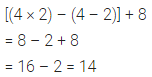Question 7.
48 + 96 – 24 – 6 x 18
Solution: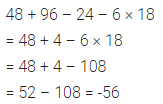Question 8.
22 – [3 – {8 – (4 + 6)}]
Solution: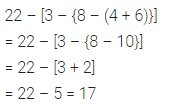Question 9.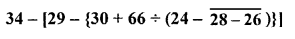Solution: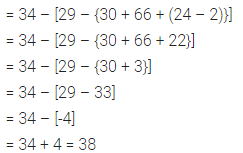Question 10.
60 – {16 + (4 x 6 – 8)}
Solution: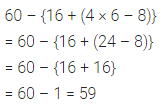Question 11.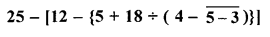Solution:Question 12.
15 – [16 – {12 + 21 ÷ (9 – 2)}]
Solution:### Playing with Numbers Exercise 9B – Selina Concise Mathematics Class 6 ICSE Solutions

Question 1.
Fill in the blanks :
(i) On dividing 9 by 7, quotient = …………. and remainder = ……….
(ii) On dividing 18 by 6, quotient = …………. and remainder = ………….
(iii) Factor of a number is ………….. of …………..
(iv) Every number is a factor of …………….
(v) Every number is a multiple of …………..
(vi) …………. is factor of every number.
(vii) For every number, its factors are ………… and its multiples are …………..
(viii) x is a factor of y, then y is a ………… of x.
Solution: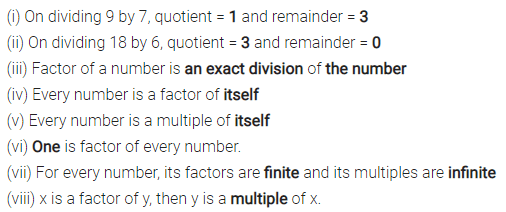Question 2.
Write all the factors of :
(i) 16
(ii) 21
(iii) 39
(iv) 48
(v) 64
(vi) 98
Solution: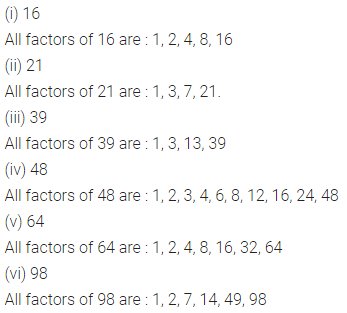Question 3.
Write the first six multiples of :
(i) 4
(ii) 9
(iii) 11
(iv) 15
(v) 18
(vi) 16
Solution: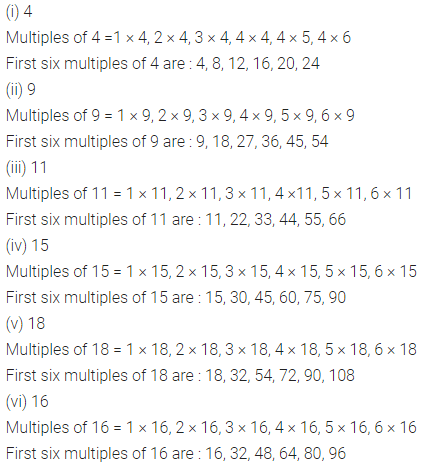Question 4.
The product of two numbers is 36 and their sum is 13. Find the numbers.
Solution: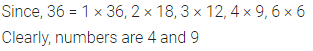Question 5.
The product of two numbers is 48 and their sum is 16. Find the numbers.
Solution: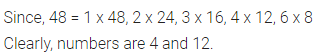Question 6.
Write two numbers which differ by 3 and whose product is 54.
Solution:Question 7.
Without making any actual division show that 7007 is divisible by 7.
Solution:Question 8.
Without making any actual division, show that 2300023 is divisible by 23.
Solution:Question 9.
Without making any actual division, show that each of the following numbers is divisible by 11.
(i) 11011
(ii) 110011
(iii) 11000011
Solution: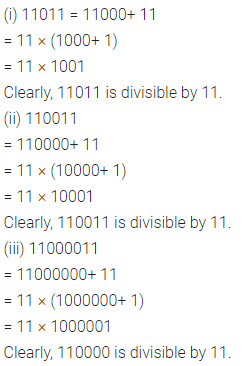Question 10.
Without actual division, show that each of the following numbers is divisible by 8 :
(i) 1608
(ii) 56008
(iii) 240008
Solution: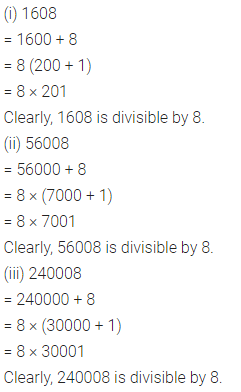### Playing with Numbers Exercise 9C – Selina Concise Mathematics Class 6 ICSE Solutions

Question 1.
find which of the following numbers are divisible by 2 :
(i) 352
(ii) 523
(iii) 496
(iv) 649
Solution: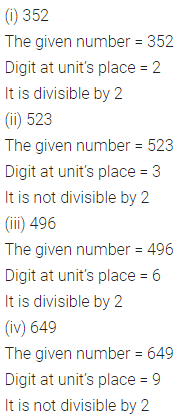Question 2.
Find which of the following number are divisible by 4 :
(i) 222
(ii) 532
(iii) 678
(iv) 9232
Solution: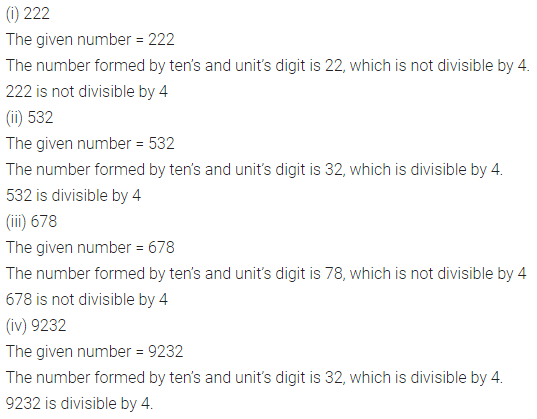Question 3.
Find the which of the following numbers are divisible by 8 :
(i) 324
(ii) 2536
(iii) 92760
(iv) 444320
Solution:Question 4.
Find which of the following numbers are divisible by 3 :
(i) 221
(ii) 543
(iii) 28492
(iv) 92349
Solution:Question 5.
Find which of the following numbers are divisible by 9 :
(i) 1332
(ii) 53247
(iii) 4968
(iv) 200314
Solution: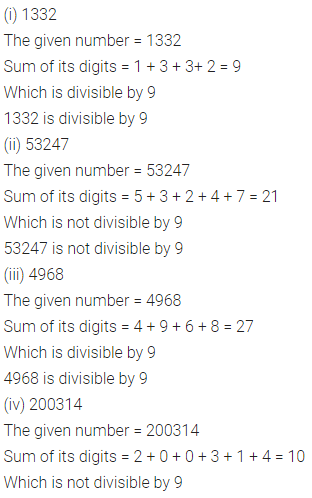Question 6.
Find which of the following number are divisible by 6 :
(i) 324
(ii) 2010
(iii) 33278
(iv) 15505
Solution: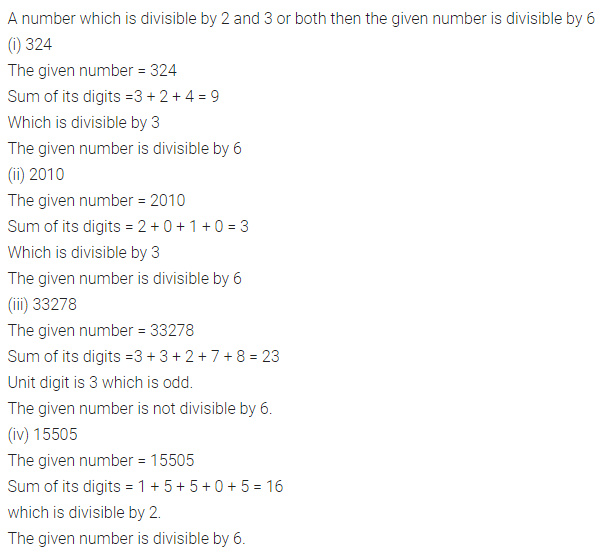Question 7.
Find which of the following numbers are divisible by 5 :
(i) 5080
(ii) 66666
(iii) 755
(iv) 9207
Solution: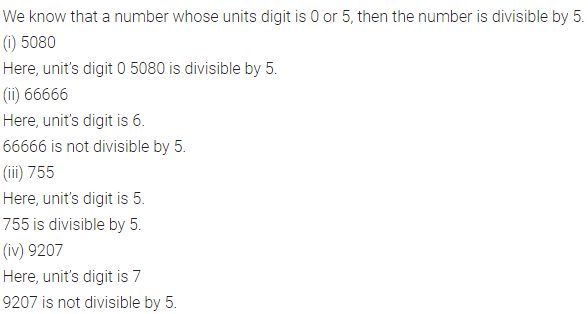Question 8.
Find which of the following numbers are divisible by 10 :
(i) 9990
(ii) 0
(iii) 847
(iv) 8976
Solution:Question 9.
Find which of the following numbers are divisible by 11 :
(i) 5918
(ii) 68,717
(iii) 3882
(iv) 10857
Solution: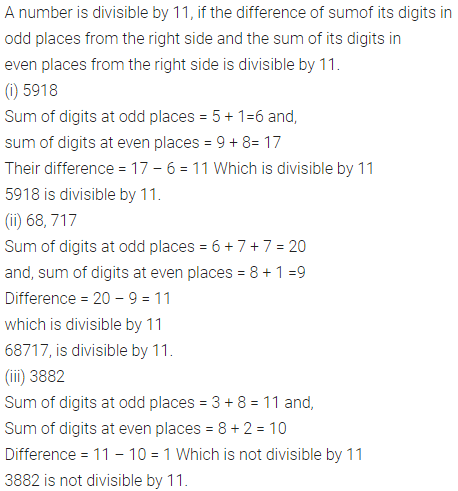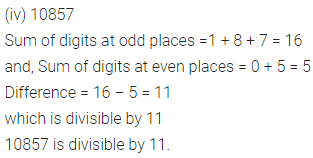Question 10.
Find which of the following numbers are divisible by 15 :
(i) 960
(ii) 8295
(iii) 10243
(iv) 5013
Solution:Question 11.
In each of the following numbers, replace M by the smallest number to make resulting number divisible by 3 :
(i) 64 M 3
(ii) 46 M 46
(iii) 27 M 53
Solution:Question 12.
In each of the following numbers replace M by the smallest number to make resulting number divisible by 9.
(i) 76 M 91
(ii) 77548 M
(iii) 627 M 9
Solution:Question 13.
In each of the following numbers, replace M by the smallest number to make resulting number divisible by 11.
(i) 39 M 2
(ii) 3 M 422
(iii) 70975 M
(iv) 14 M 75
Solution: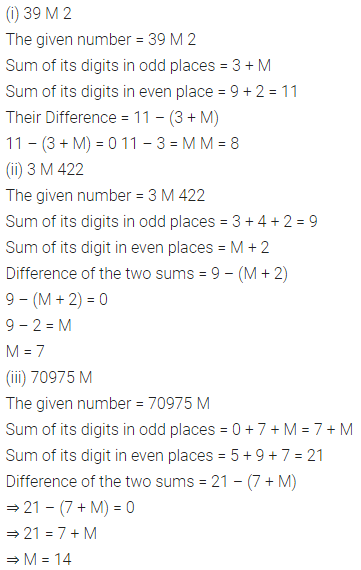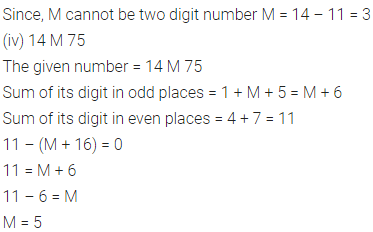Question 14.
State, true or false :
(i) If a number is divisible by 4. It is divisible by 8.
(ii) If a number is a factor of 16 and 24, it is a factor of 48.
(iii) If a number is divisible by 18, it is divisible by 3 and 6.
(iv) If a divide b and c completely, then a divides (i) a + b (ii) a – b also completely.
Solution: